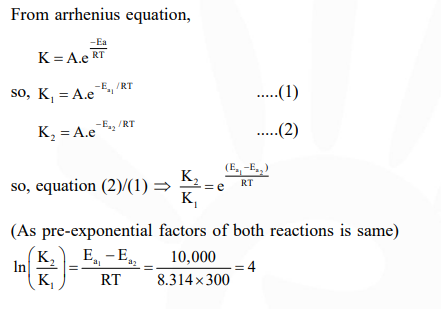# Two reactions

Question:

Two reactions $R_{1}$ and $R_{2}$ have identical pre-exponential factors. Activation energy of $R_{1}$ exceeds that of $\mathrm{R}_{2}$ by $10 \mathrm{~kJ} \mathrm{~mol}^{-1}$. If $\mathrm{k}_{1}$ and $\mathrm{k}_{2}$ are rate constants for reactions $\mathrm{R}_{1}$ and $\mathrm{R}_{2}$ respectively at $300 \mathrm{~K}$, then $\ln \left(\mathrm{k}_{2} / \mathrm{k}_{1}\right)$ is equal to :-

$\left(\mathrm{R}=8.314 \mathrm{~J} \mathrm{~mol}^{-1} \mathrm{~K}^{-1}\right)$

1. 8

2. 12

3. 6

4. 4

Correct Option: , 4

Solution: